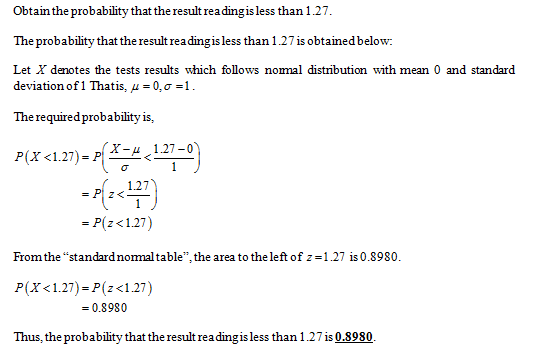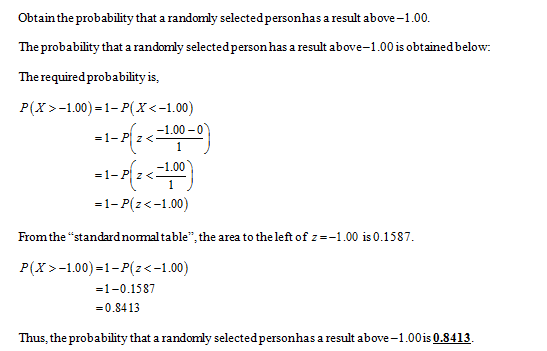# The population of z scores is normally distributed with a mean of 0 and a standard deviation of 1, so these test results meet the requirements of a standard normal deviation;a) A randomly selected adult undergoes a bone density test, find the probabliity that the result is a reading less than 1.27.b)Find the probability that a randomly selected person has a result above -1.00. A value above -1.00 is considered to be in the "normal" range of bone density readings.c) A bone density test reading between -1.00 and -2.50 indicates that the subject has osteopenia, which is some bone loss. Find the probability that the randomly selected subject has a reading between -1.00 and -2.50.

Question
2 views

The population of z scores is normally distributed with a mean of 0 and a standard deviation of 1, so these test results meet the requirements of a standard normal deviation;

a) A randomly selected adult undergoes a bone density test, find the probabliity that the result is a reading less than 1.27.

b)Find the probability that a randomly selected person has a result above -1.00. A value above -1.00 is considered to be in the "normal" range of bone density readings.

c) A bone density test reading between -1.00 and -2.50 indicates that the subject has osteopenia, which is some bone loss. Find the probability that the randomly selected subject has a reading between -1.00 and -2.50.

check_circle

Part aPart b...

### Want to see the full answer?

See Solution

#### Want to see this answer and more?

Solutions are written by subject experts who are available 24/7. Questions are typically answered within 1 hour.*

See Solution
*Response times may vary by subject and question.
Tagged in